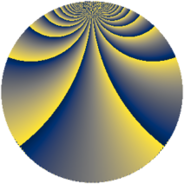# Properties

 Label 2304.3.mLevel $2304$ Weight $3$ Character orbit 2304.m Rep. character $\chi_{2304}(703,\cdot)$ Character field $\Q(\zeta_{4})$ Dimension $160$ Sturm bound $1152$

# Related objects

## Defining parameters

 Level: $$N$$ $$=$$ $$2304 = 2^{8} \cdot 3^{2}$$ Weight: $$k$$ $$=$$ $$3$$ Character orbit: $$[\chi]$$ $$=$$ 2304.m (of order $$4$$ and degree $$2$$) Character conductor: $$\operatorname{cond}(\chi)$$ $$=$$ $$16$$ Character field: $$\Q(i)$$ Sturm bound: $$1152$$

## Dimensions

The following table gives the dimensions of various subspaces of $$M_{3}(2304, [\chi])$$.

Total New Old
Modular forms 1632 160 1472
Cusp forms 1440 160 1280
Eisenstein series 192 0 192

## Trace form

 $$160q + O(q^{10})$$ $$160q + 1120q^{49} - 192q^{65} + O(q^{100})$$

## Decomposition of $$S_{3}^{\mathrm{new}}(2304, [\chi])$$ into newform subspaces

The newforms in this space have not yet been added to the LMFDB.

## Decomposition of $$S_{3}^{\mathrm{old}}(2304, [\chi])$$ into lower level spaces

$$S_{3}^{\mathrm{old}}(2304, [\chi]) \cong$$ $$S_{3}^{\mathrm{new}}(16, [\chi])$$$$^{\oplus 15}$$$$\oplus$$$$S_{3}^{\mathrm{new}}(48, [\chi])$$$$^{\oplus 10}$$$$\oplus$$$$S_{3}^{\mathrm{new}}(64, [\chi])$$$$^{\oplus 9}$$$$\oplus$$$$S_{3}^{\mathrm{new}}(128, [\chi])$$$$^{\oplus 6}$$$$\oplus$$$$S_{3}^{\mathrm{new}}(144, [\chi])$$$$^{\oplus 5}$$$$\oplus$$$$S_{3}^{\mathrm{new}}(192, [\chi])$$$$^{\oplus 6}$$$$\oplus$$$$S_{3}^{\mathrm{new}}(256, [\chi])$$$$^{\oplus 3}$$$$\oplus$$$$S_{3}^{\mathrm{new}}(384, [\chi])$$$$^{\oplus 4}$$$$\oplus$$$$S_{3}^{\mathrm{new}}(576, [\chi])$$$$^{\oplus 3}$$$$\oplus$$$$S_{3}^{\mathrm{new}}(768, [\chi])$$$$^{\oplus 2}$$$$\oplus$$$$S_{3}^{\mathrm{new}}(1152, [\chi])$$$$^{\oplus 2}$$